Next: Solving Equation of Motion Up: Physics and Numerical Methods Previous: Description of Polarization

## Beam Parameters

The BEAM command makes it possible to define a beam in terms of the conventional Twiss parameters. A beam is defined by many parameters described in Sec.3.3. Here, we will give formulas how to generate a beam using these parameters. An important point is that the beam is defined on a plane s=constant rather than t=constant. Thus, the longitudinal structure of the beam appears as the time structure. Note that t is larger at the bunch tail.

The parameters are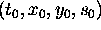Reference point of the Twiss parameters. (m)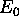Reference energy. (eV)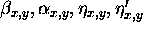Twiss parameters.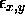Geometric emittance. (radm)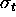R.m.s. bunch length. (m)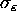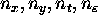Gaussian tail cut off.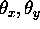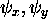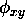Beam roll in the x-y plane. (rad)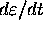Coherent energy slope (1/m).

First, generate particle variables in usual accelerator coordinate: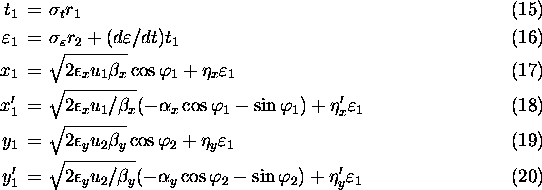where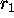(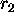) is a Gaussian random number of zero mean and unit standard deviation cut at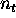(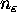),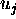(j=1,2) is a random number of exponential distribution (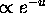) cut at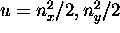and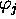a uniform random number in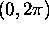.

These variables are then transformed to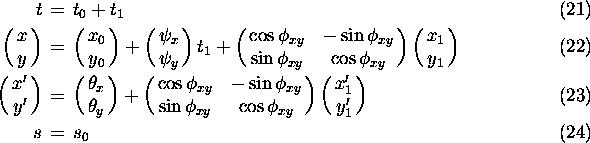Finally, the energy-momentum is given by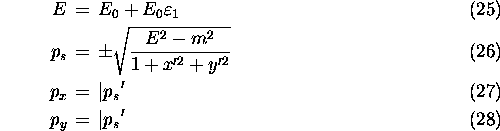where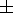is + for right-going beam and - for left-going (note that right/left appears only here) and m is the relevant particle mass in units of eV/c.

Toshiaki Tauchi
Thu Dec 3 17:27:26 JST 1998